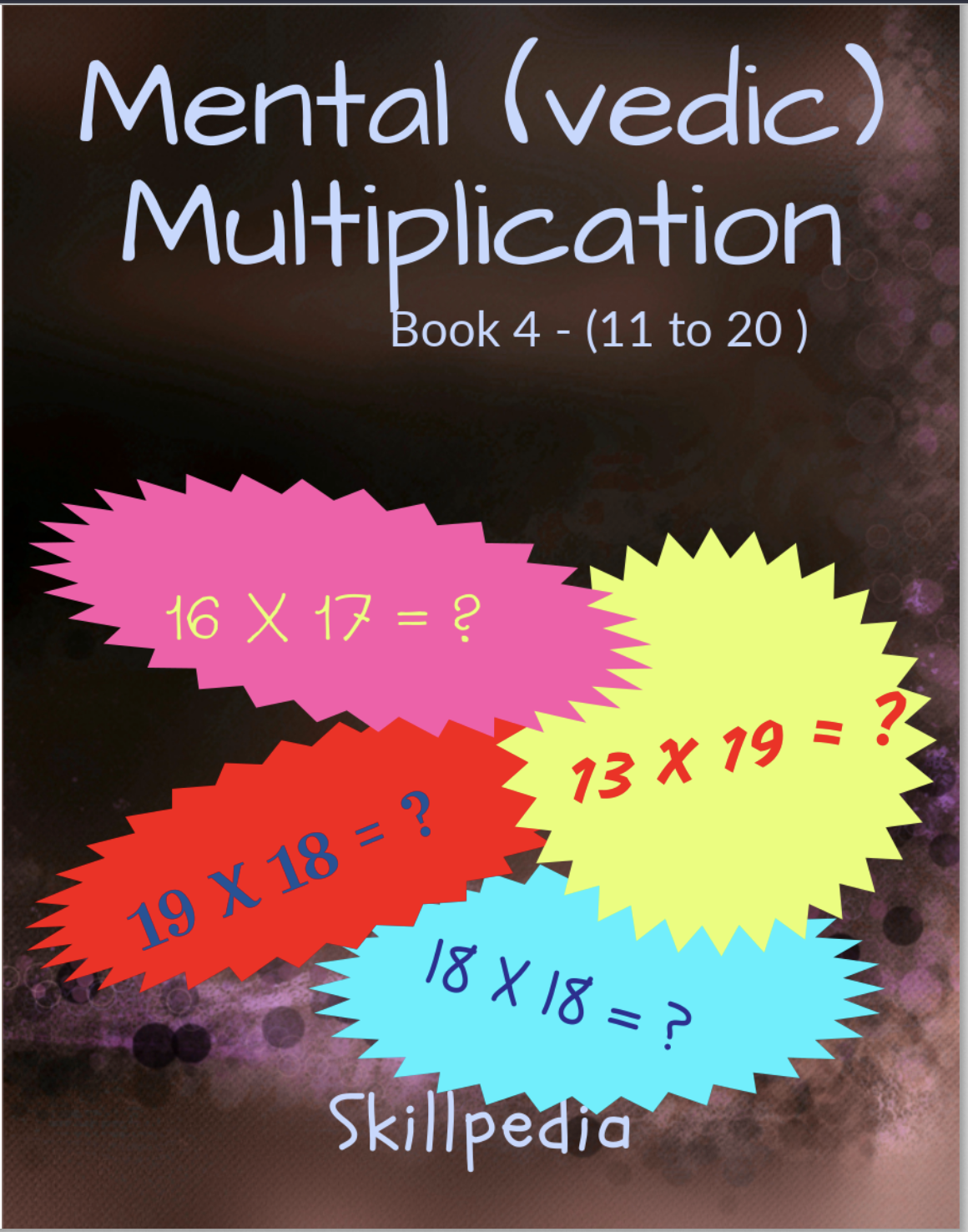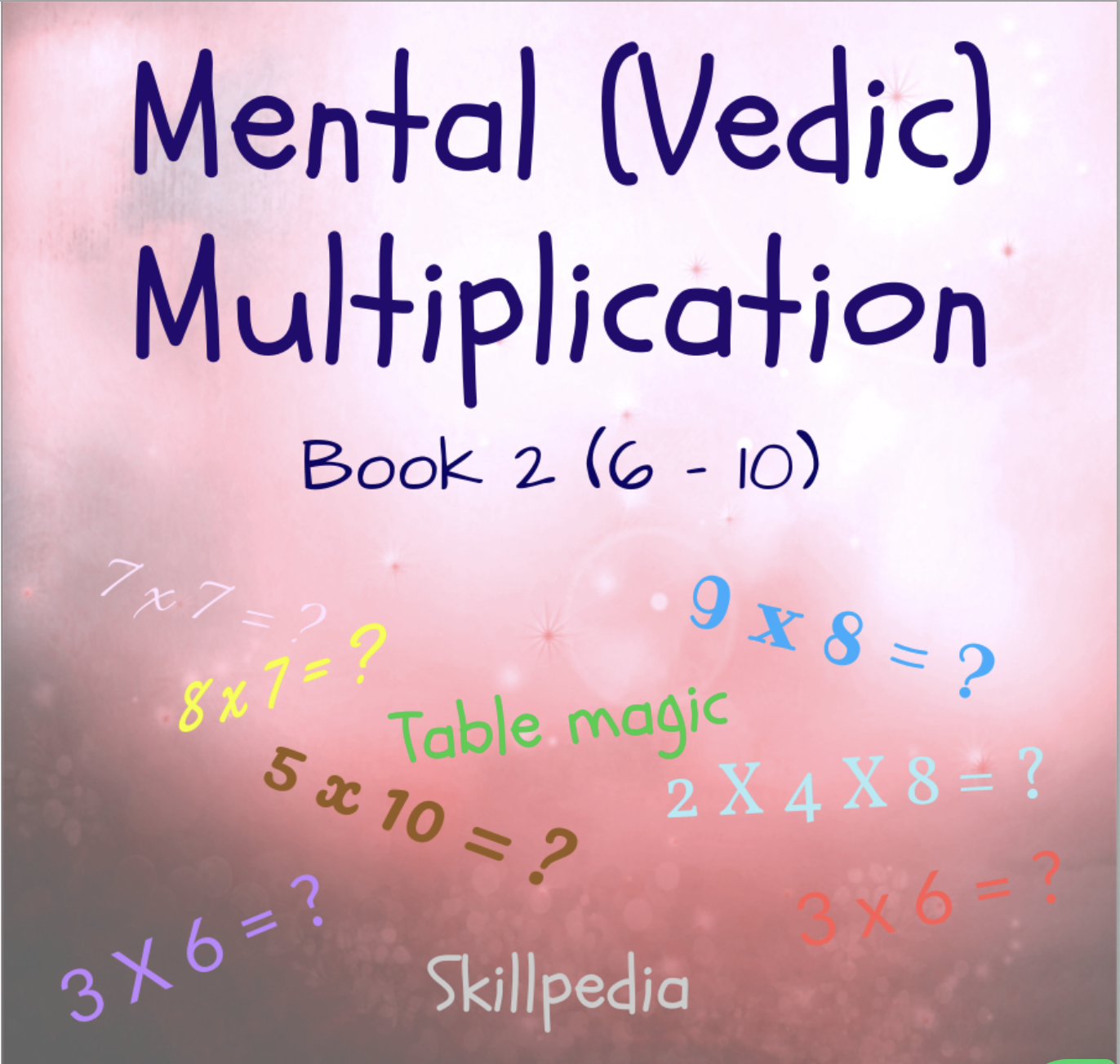### All Resources

Showing 13 to 24 of 106 product(s)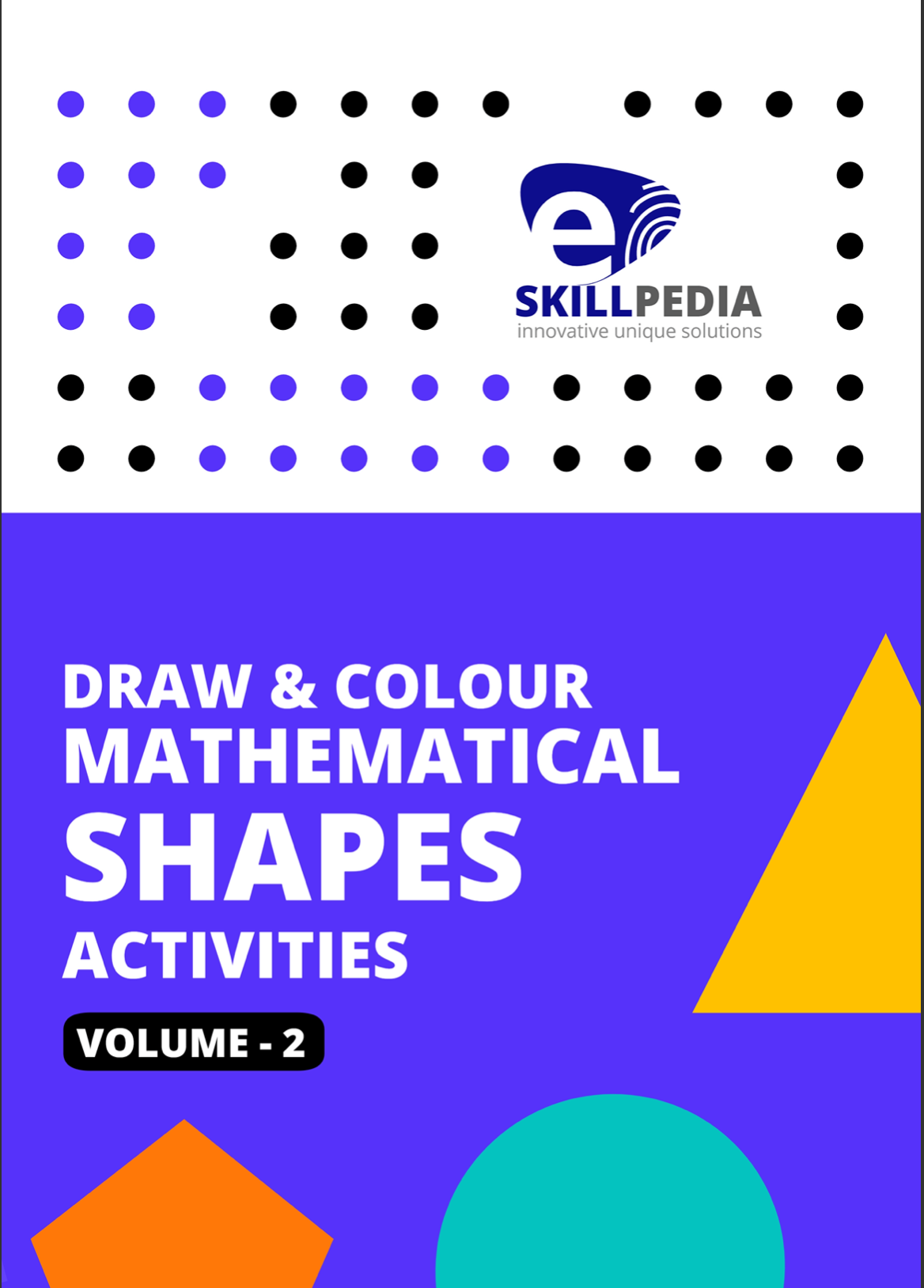### 13. Draw & Colour Mathematical Shapes Activities (Volume 2)

Draw & Colour Mathematical Shapes (Volume - 2) This

\$2.0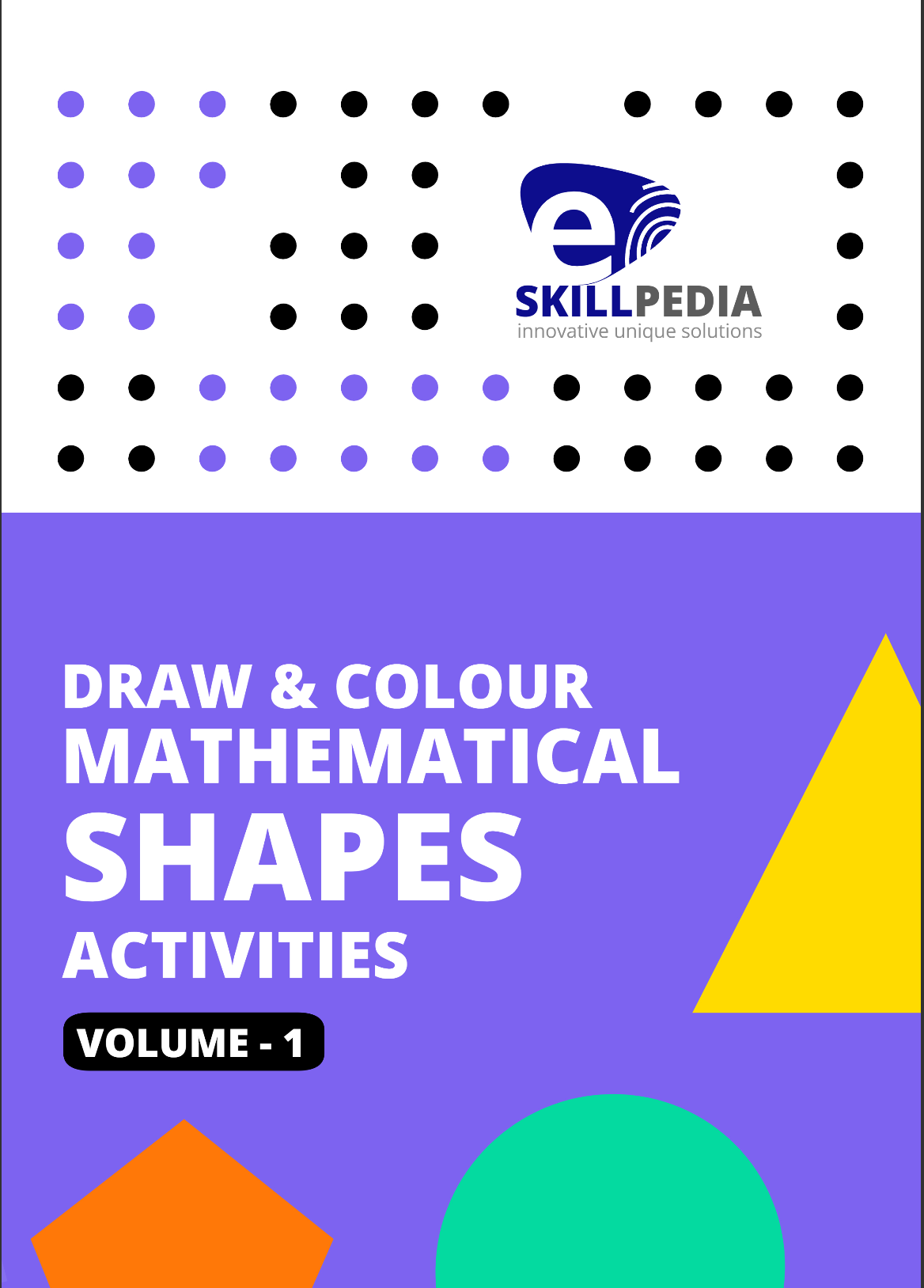### 14. Draw & Colour Mathematical Shapes Activities (Volume - 1)

Draw & Colour Mathematical Shapes (Volume - 1) A

\$2.0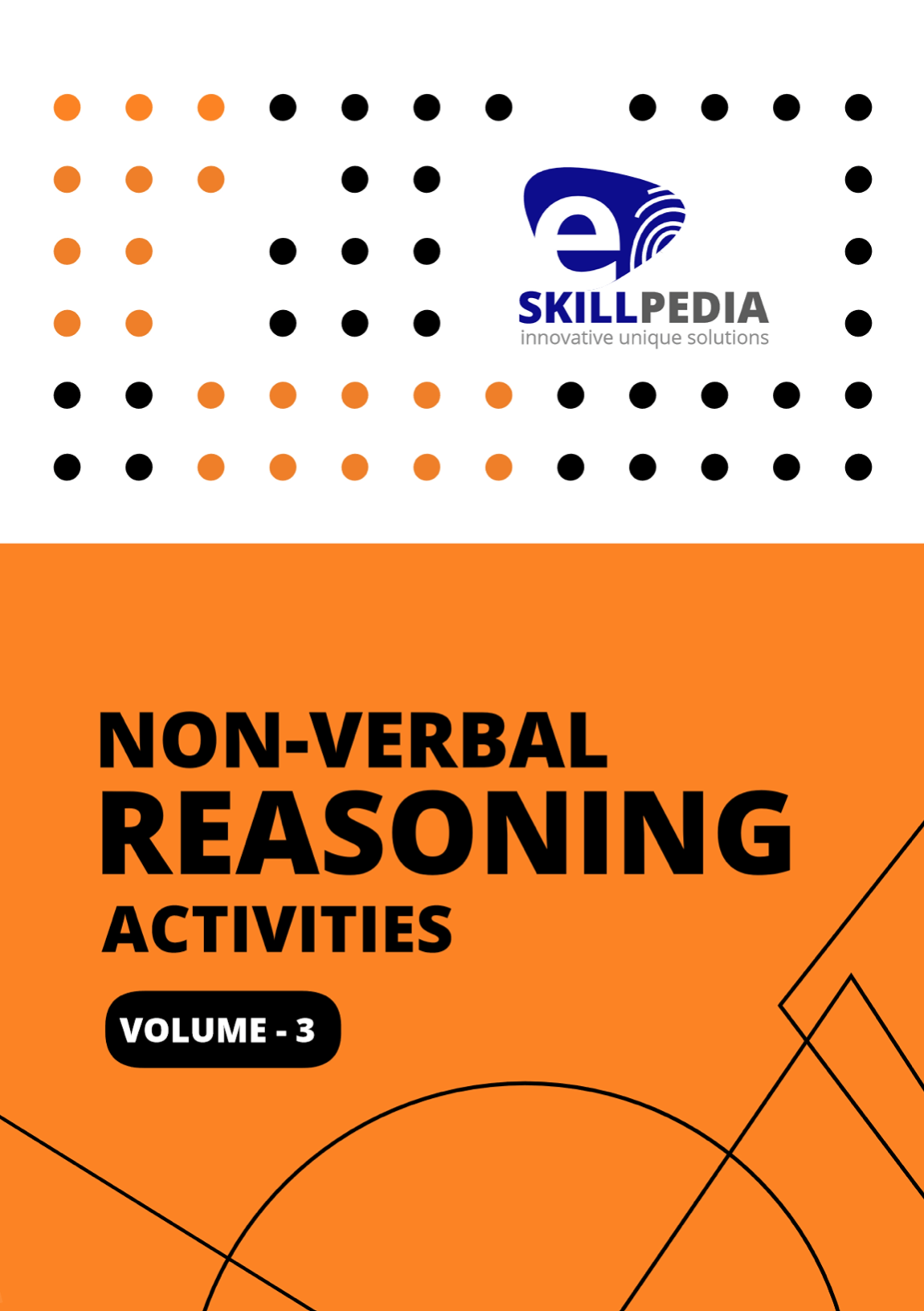### 15. Non-Verbal Reasoning Activities (Volume 3)

Non-Verbal Reasoning (Volume - 3) Advanced Non-verbal

\$2.0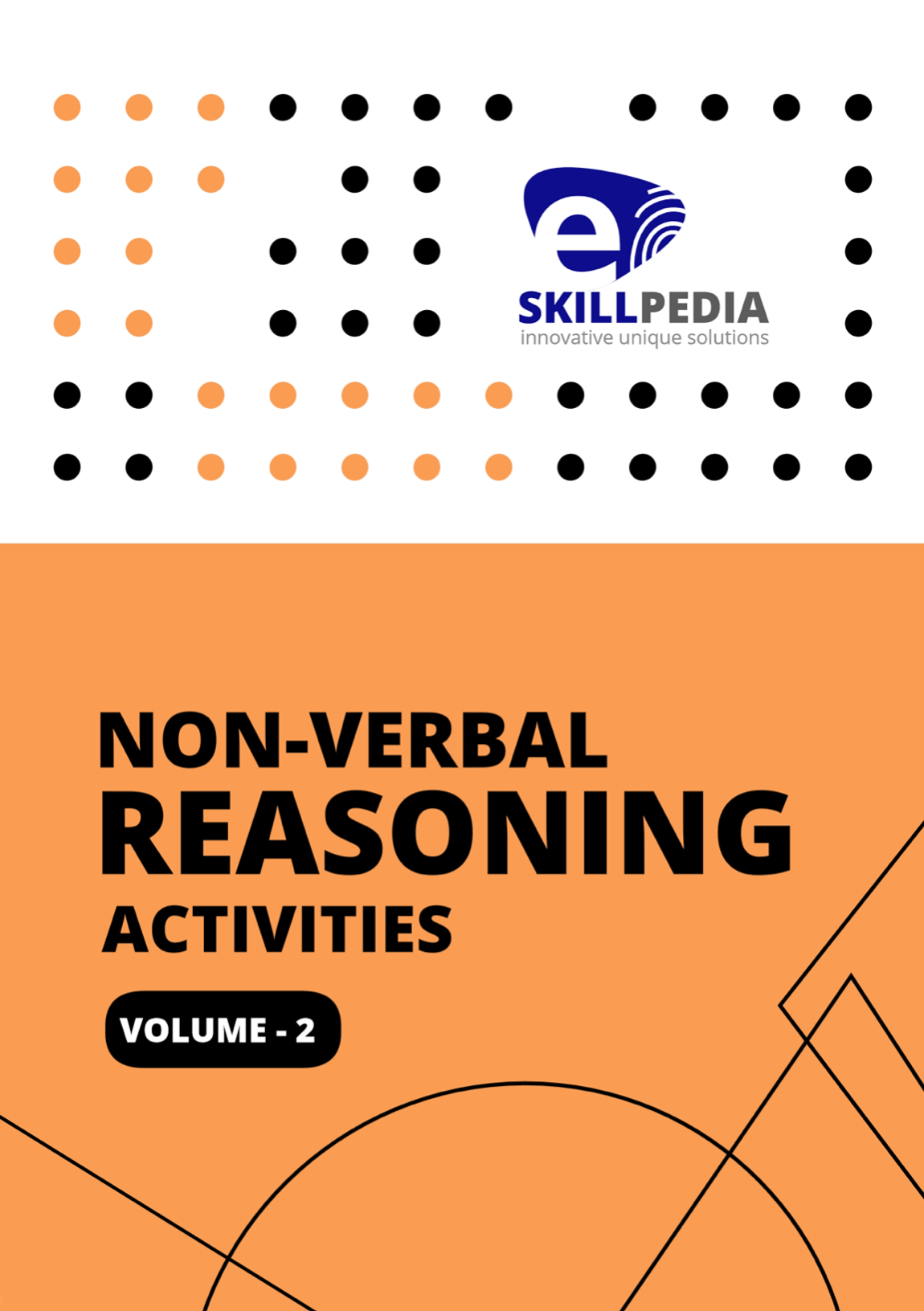### 16. Non-Verbal Reasoning Activities (Volume 2)

Non-Verbal Reasoning - (Volume 2) The questions have

\$2.0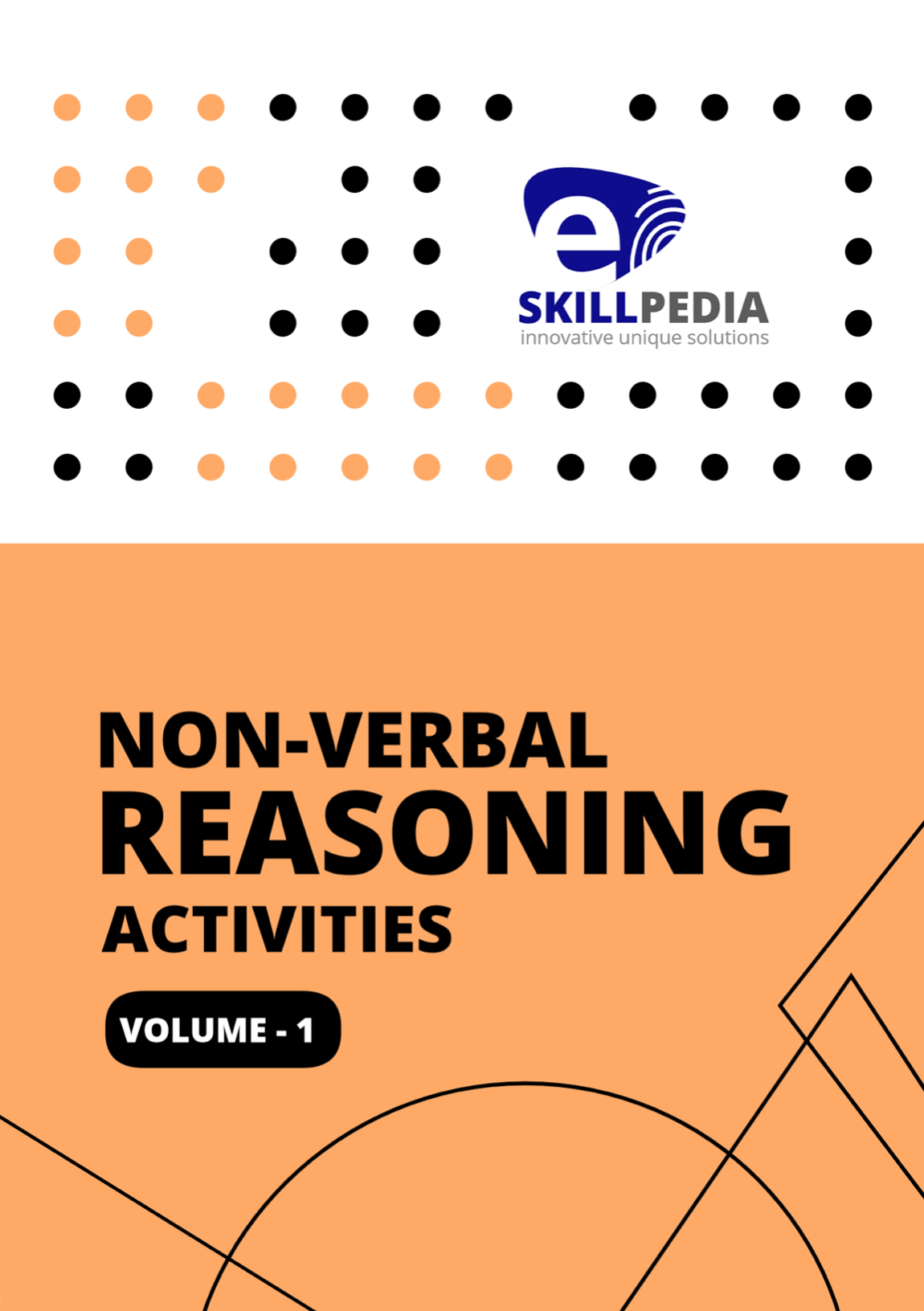### 17. Non-verbal Reasoning Activities (Volume 1)

Non-Verbal Reasoning - Volume 1 This bok deals with

\$2.0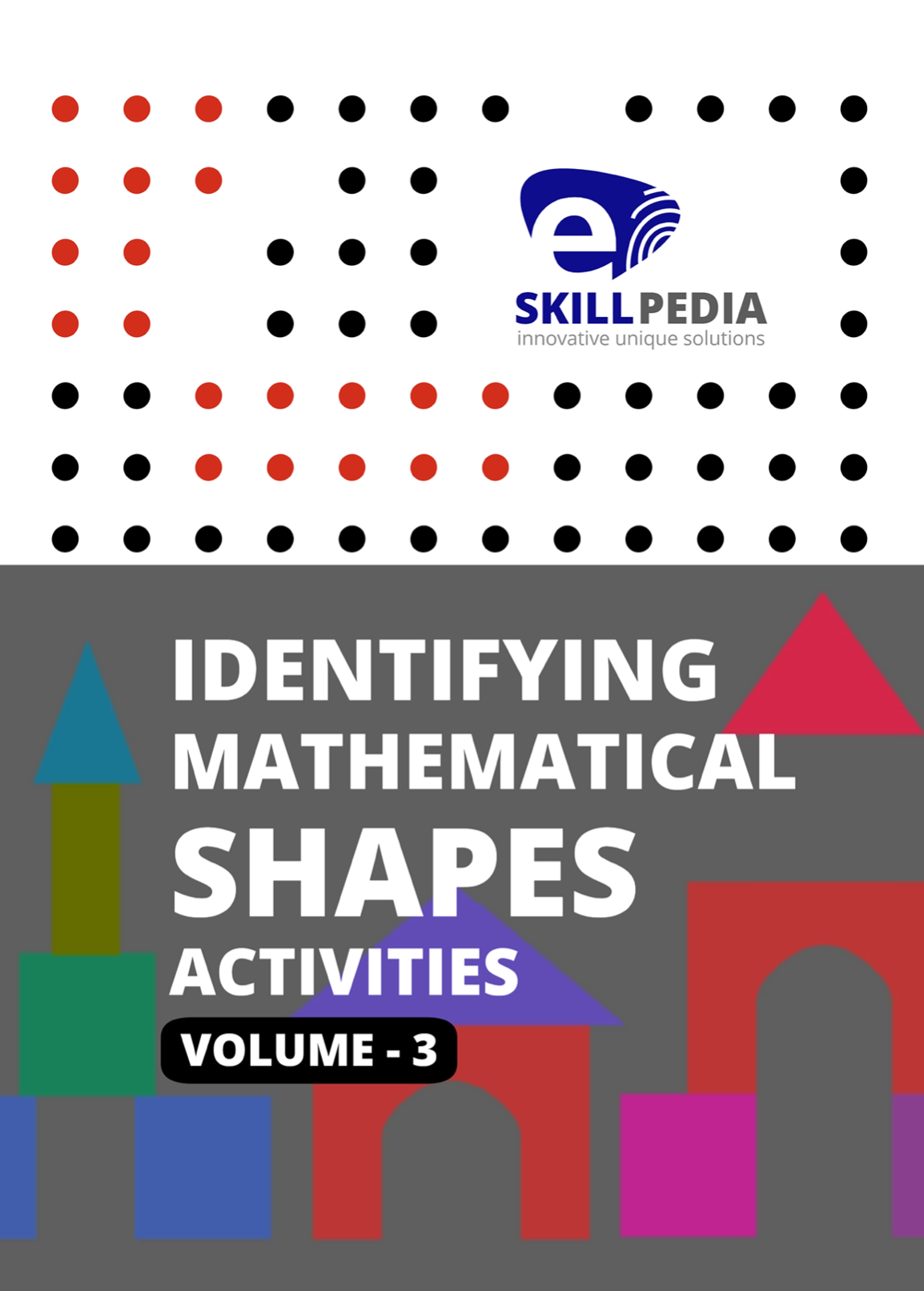### 18. Identifying Mathematical Shapes Activities (Volume 3)

Identifying Mathematical Shapes  In this volume we focus about construct architect buildings using shapes,

\$2.0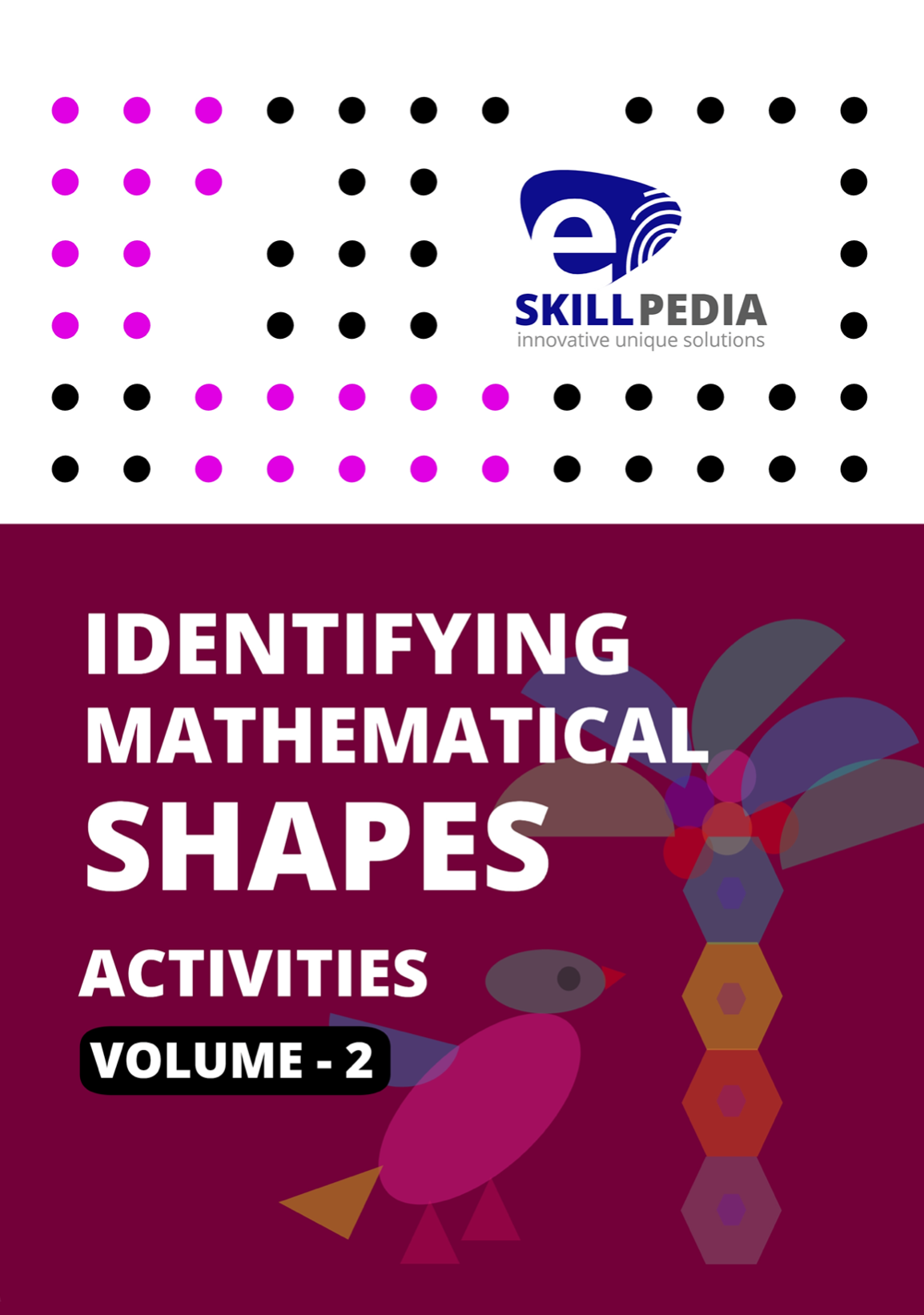### 19. Identifying Mathematical Shapes Activities (Volume 2)

Identifying MAthematical Shapes In this volume kids

\$2.0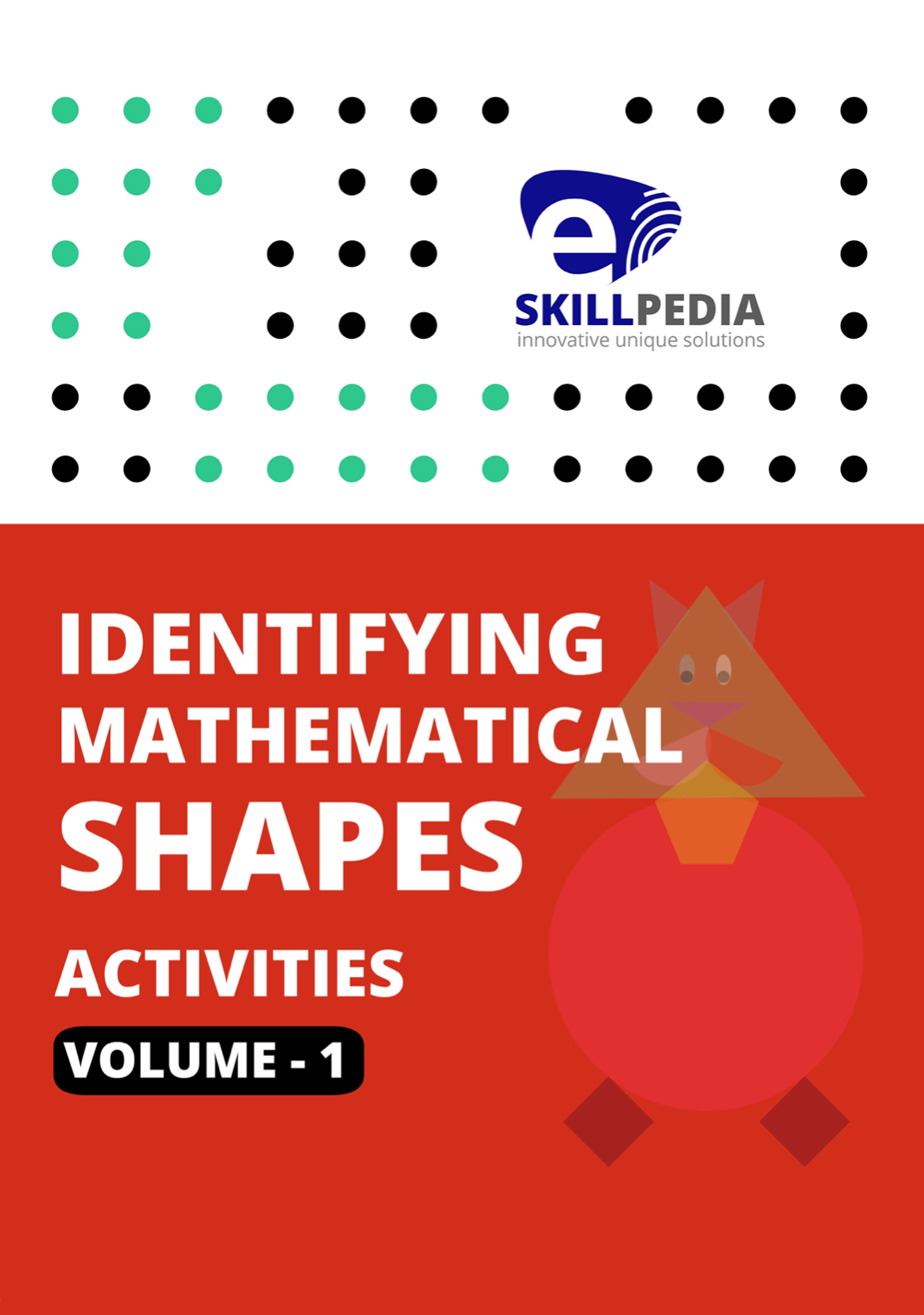### 20. Identifying Mathematical Shapes (Volume 1)

Identifying Mathematical Shapes  Identifying shapes is one of the favorite student activities. In

\$2.0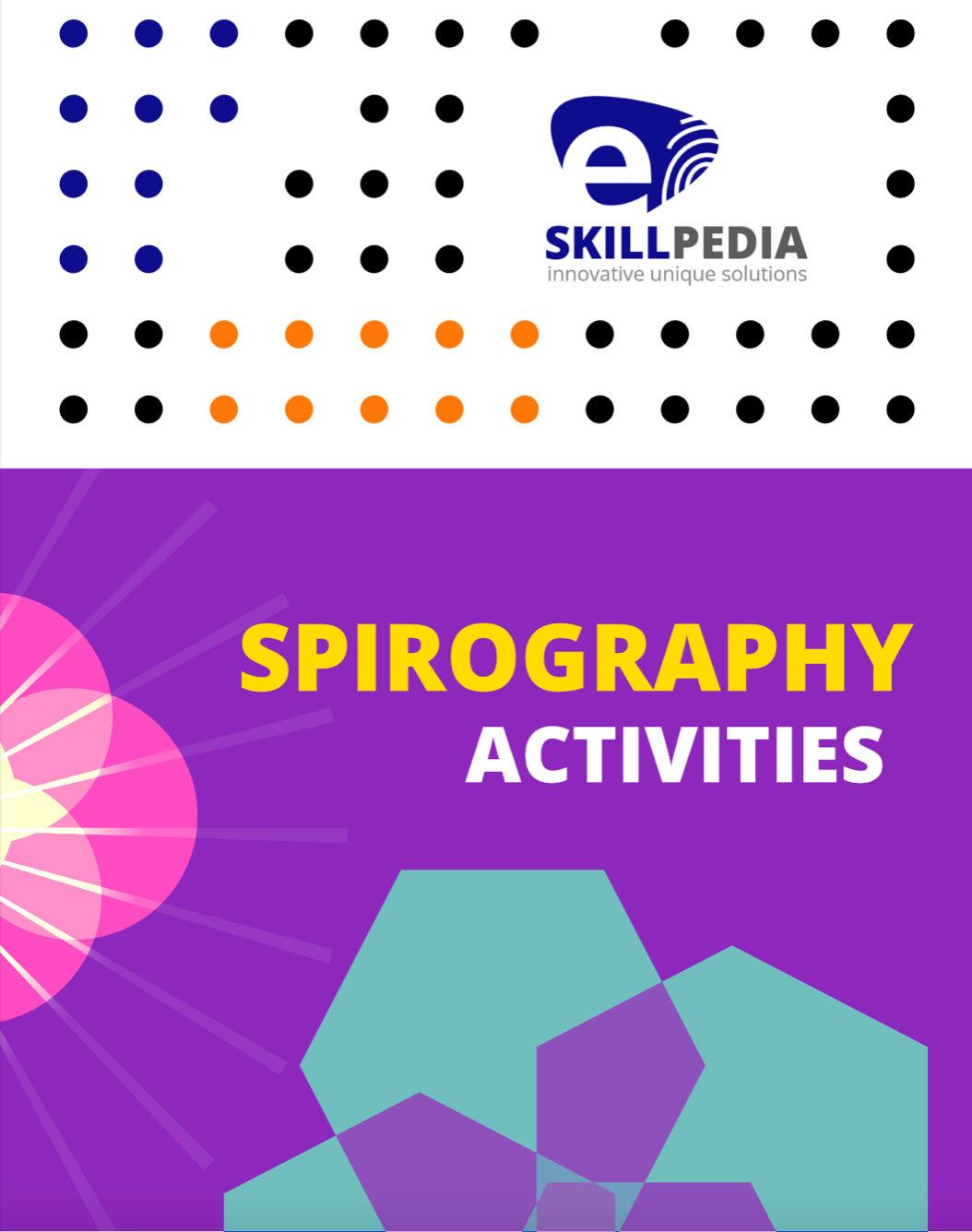### 21. Spirograph

This post is about how to draw Spirograph pattern art with ruler and marker.